Tuesday, September 26, 2023

# Get started with NOT Gate IC | 7400 Series Tutorials

Logic gates are the basic building blocks of digital electronics. More common ones being the OR, AND, NOR, NAND, EX-OR and NOT gates. Typically, a gate takes input from two input terminals, performs some operation and outputs the result at an output terminal. The NOT gate, however lacks the second input. It consists of only one input and one output. A NOT gate is used to output the Boolean inverse of the applied input. Hence the name ‘Digital Inverter’ or ‘Inverting buffer’. The most commonly used NOT gate IC is the 7404.

## NOT Gate Logic Symbol and Boolean Expression

A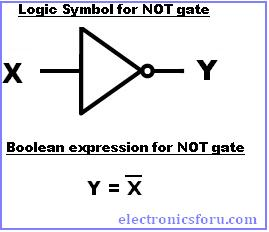s NOT gate IC is an inverter, the output is represented by a “ ͞ “ (bar) symbol over the input. The Boolean expression can be written as Y = X͞. That is, if X is the input and Y is output, then output Y equals to X͞, not X. In other words, when X=0, Y =1 and vice versa.
The bubble in the symbol for a NOT gate at the output port represents the inverting operation. That means for a high logic signal input, the output of the NOT gate IC will be LOW. Similarly for LOW logic signal input, the output of NOT gate IC will be HIGH.

## Truth Table for NOT gate IC

“If X is NOT true, then Y is true”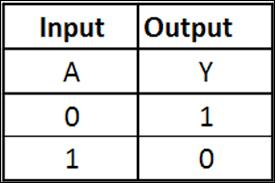## Recommended operating conditions of NOT gate IC 7404

Recommended operating conditions of 7404 NOT gate IC are given in the following table. Ideally, the supply voltage should range between 5.25V and 4.75V. A voltage greater than 2V will be considered as HIGH (1) and voltage less than 0.8V will be considered as LOW (0).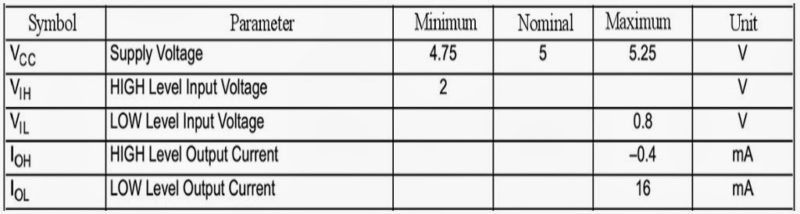## Pin-out diagram of 7404 NOT gate IC

Each 7404 NOT gate IC has 6 NOT gates arranged as shown in the following figure. 14th pin is the Vcc and the 7th pin is the Ground. The outputs directly interface to CMOS, NMOS and TTL. It supports wide operating conditions and has large operating voltage range.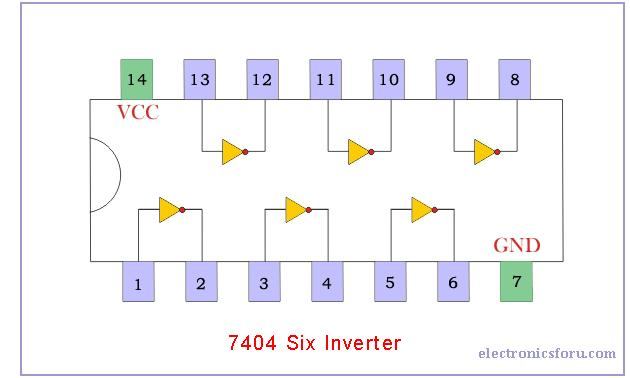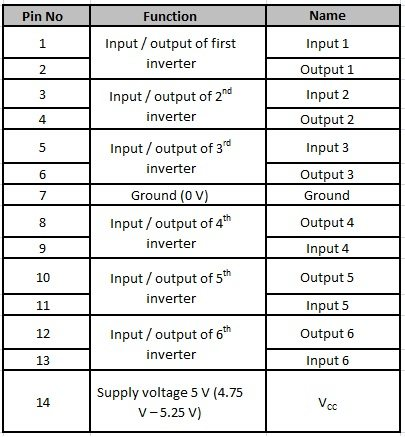The datasheet for NOT gate IC 7404 is available at

NOT gate is however just on of the 7400 series. For the 7400 series tutorial, turn over to next page.